# why m=1, and why n=pi/2, 3pi/2, ............ The problem of unsteady heat conduction in a metal...

why m=1, and why n=pi/2, 3pi/2, ............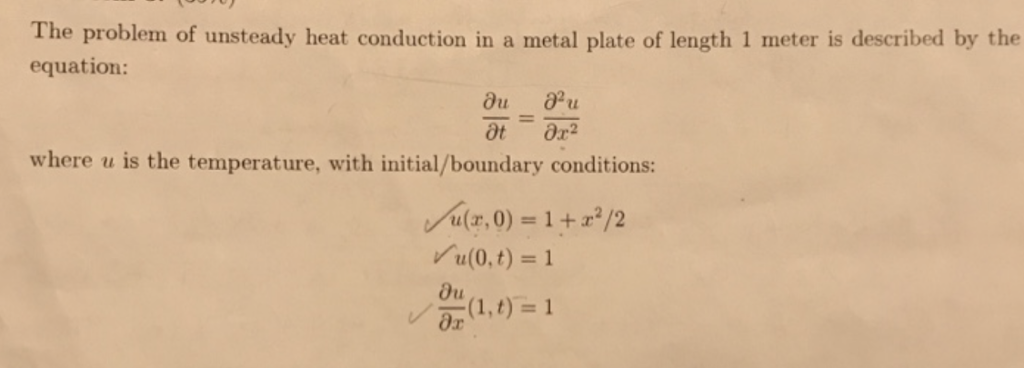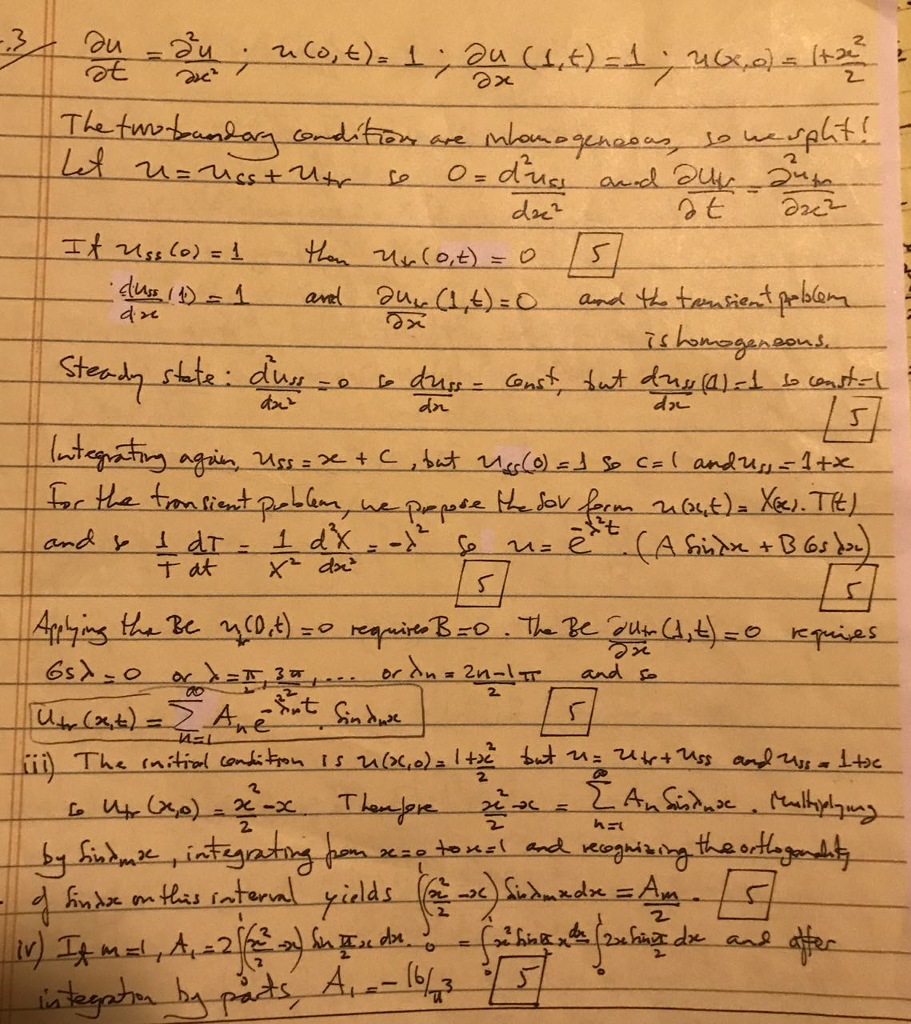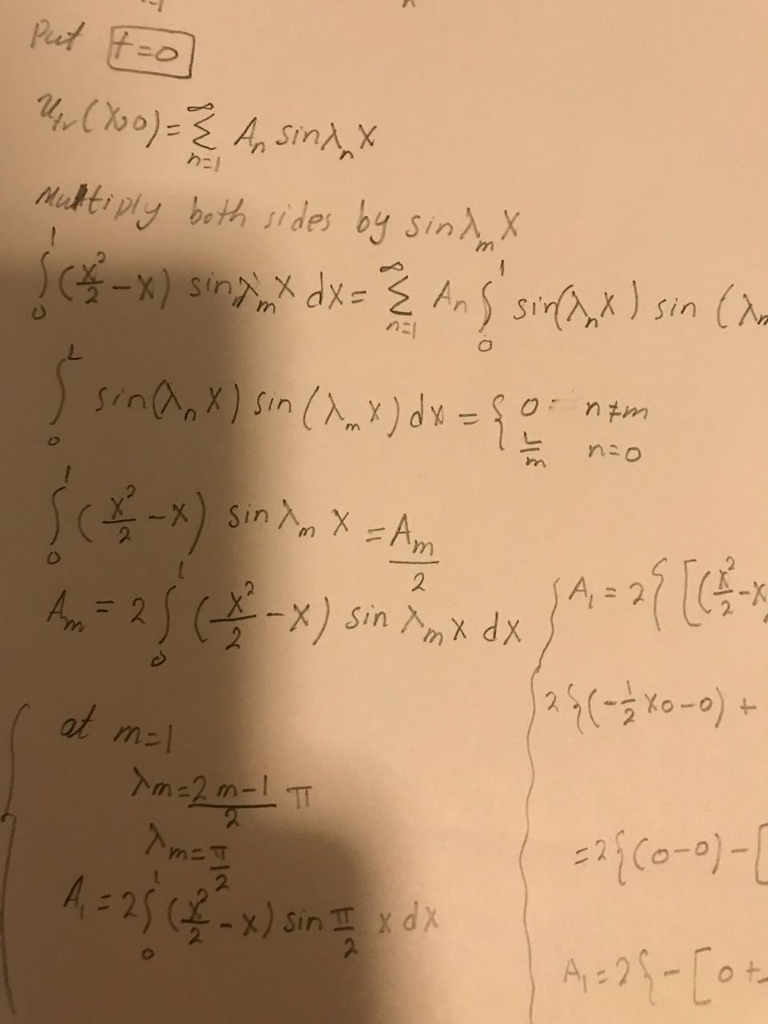The problem of unsteady heat conduction in a metal plate of length 1 meter is described by the equation: where u is the temperature, with initial/boundary conditions: a(2,0) = 1 (0,t) = 1 Ou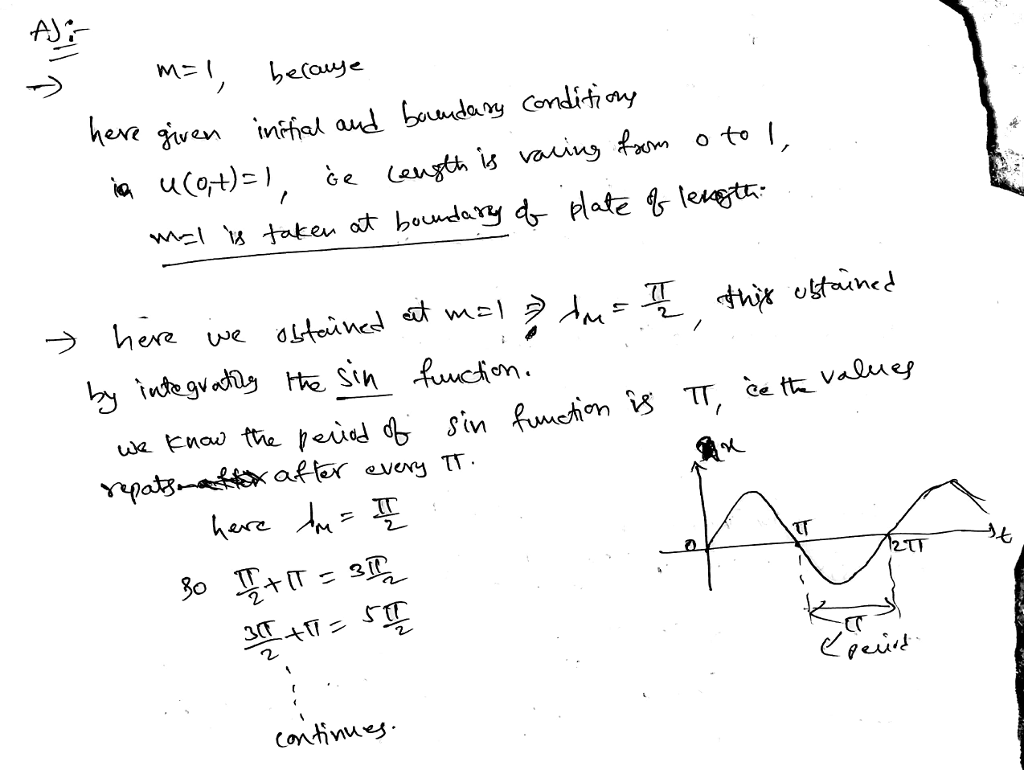#### Earn Coin

Coins can be redeemed for fabulous gifts.

Similar Homework Help Questions
• ### Problem 3 Solve the unsteady heat conduction problem: subject to the boundary conditions: u(0,t)0, (1,t)1; and...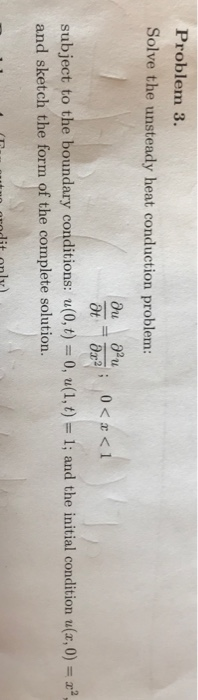Problem 3 Solve the unsteady heat conduction problem: subject to the boundary conditions: u(0,t)0, (1,t)1; and the initial condition ua, 0) and sketch the form of the complete solution.

• ### Heat is supplied to the exposed surface. Assume unidirectional unsteady state heat conduction according to the...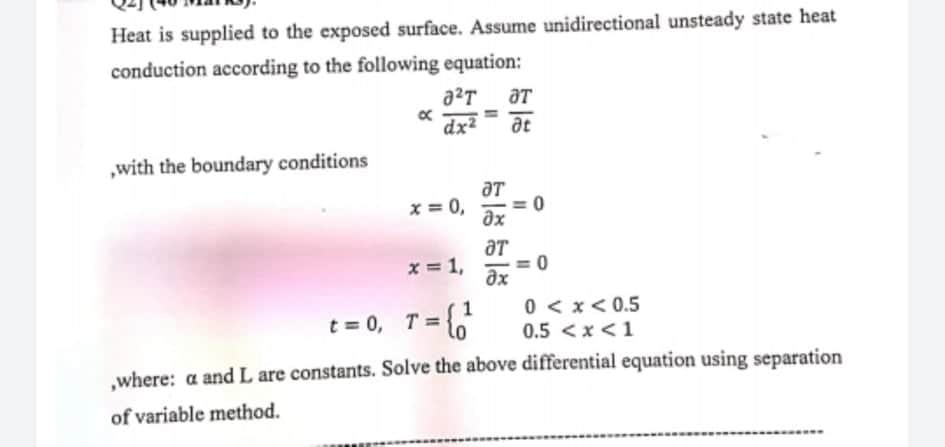Heat is supplied to the exposed surface. Assume unidirectional unsteady state heat conduction according to the following equation: a?T at dx² ac with the boundary conditions ат x = 0, дх ат x = 1, 0 дх 0 < x < 0.5 t = 0, T= {. 0.5 <x<1 ,where: a and L are constants. Solve the above differential equation using separation of variable method.

• ### 3. In class we discussed the heat conduction problem with the boundary conditions a(0, t) 0, t4(1...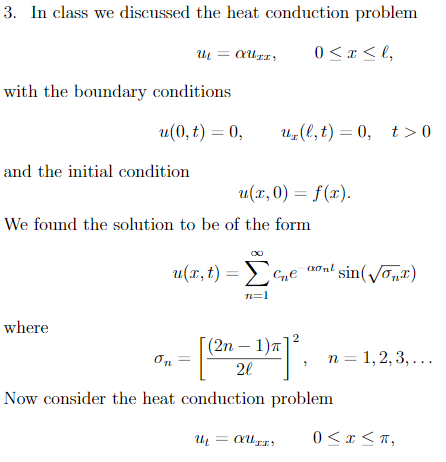3. In class we discussed the heat conduction problem with the boundary conditions a(0, t) 0, t4(1,t)-0, t > 0 and the initial condition u(r,0) f(a) We found the solution to be of the form where (2n-1)n 1,2,3,. TL 20 Now consider the heat conduction problem with the boundary conditions u(0, t) 1,u(T, t)0, t>0 and the initial condition ur,0) 0. Find u(r,t). Hint: First you must find the steady state. 3. In class we discussed the heat conduction problem...

• ### Consider a 2 m long metal rod. The temperature u(z,t) at a point along the rod at any time t is found by solving the heat equation k where k is the material property. The left end of the rod ( 0) is...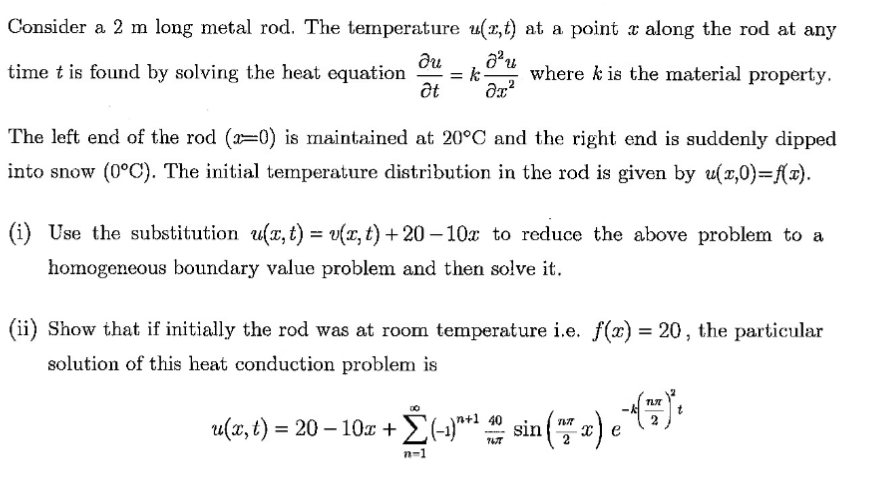Consider a 2 m long metal rod. The temperature u(z,t) at a point along the rod at any time t is found by solving the heat equation k where k is the material property. The left end of the rod ( 0) is maintained at 20°C and the right end is suddenly dipped into snow (0°C). The initial temperature distribution in the rod is given by u(x,0)- (i) Use the substitution u(z,t) ta,t)+20-10z to reduce the above problem to a...

• ### Problem 3 Using Fourier series expansion, solve the heat conduction equation in one dimension a?т ат...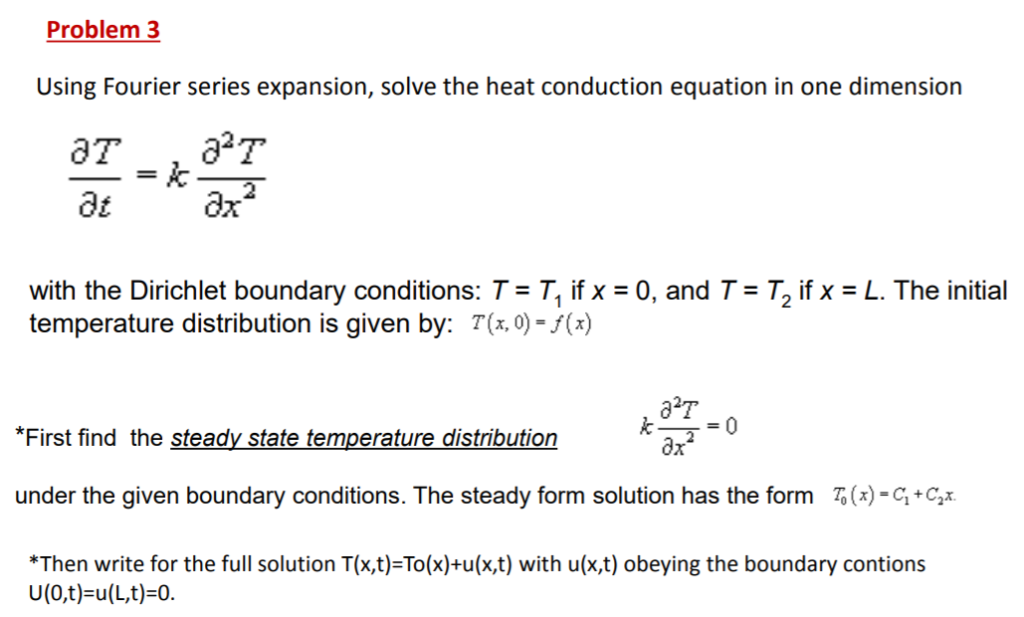Problem 3 Using Fourier series expansion, solve the heat conduction equation in one dimension a?т ат ax2 де with the Dirichlet boundary conditions: T T, if x 0, and T temperature distribution is given by: T(x, 0) -f(x) T, if x L. The initial 0 = *First find the steady state temperature distribution under the given boundary conditions. The steady form solution has the form (x)-C+C2x *Then write for the full solution T(x,t)=To(x)+u(x,t) with u(x,t) obeying the boundary contions U(0,t)...

• ### (a) Consider the one-dimensional heat equation for the temperature u(x, t), Ou,02u where c is the...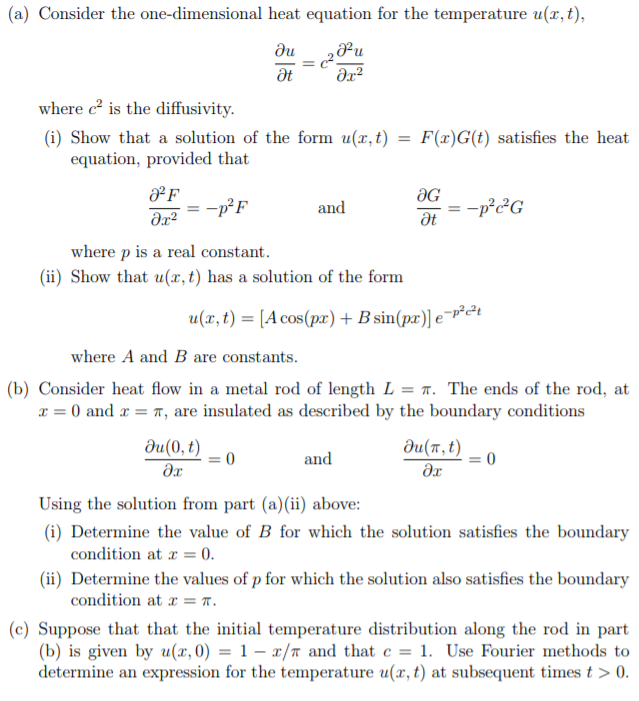(a) Consider the one-dimensional heat equation for the temperature u(x, t), Ou,02u where c is the diffusivity (i) Show that a solution of the form u(x,t)-F )G(t) satisfies the heat equation, provided that 护F and where p is a real constant (ii) Show that u(x,t) has a solution of the form (,t)A cos(pr)+ Bsin(p)le -P2e2 where A and B are constants (b) Consider heat flow in a metal rod of length L = π. The ends of the rod, at...

• ### 1. (a) Reduce the boundary value problem for 3D heat conduction with spherical symmetry u(z, y, 2...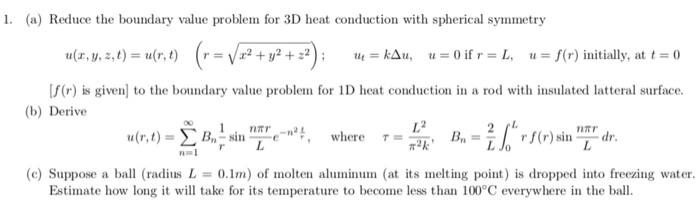PDE’s 1. (a) Reduce the boundary value problem for 3D heat conduction with spherical symmetry u(z, y, 2, t-u(r, t f(r) is given] to the boundary value problem for 1D heat conduction in a rod with insulated latteral surface (b) Derive し2 u(r, t) = Σ Bn sin nm-e-n2 ' , where Bn=2 ,rf(r) sin nTI, dr. τ= (c) Suppose a ball (radius L-0.1) of molten aluminum (at its melting point) is dropped into freezing water Estimate how long it...

• ### Q2 Given the following heat conduction initial-boundary value problem of a thin homogeneous rod, where ?(?,?)...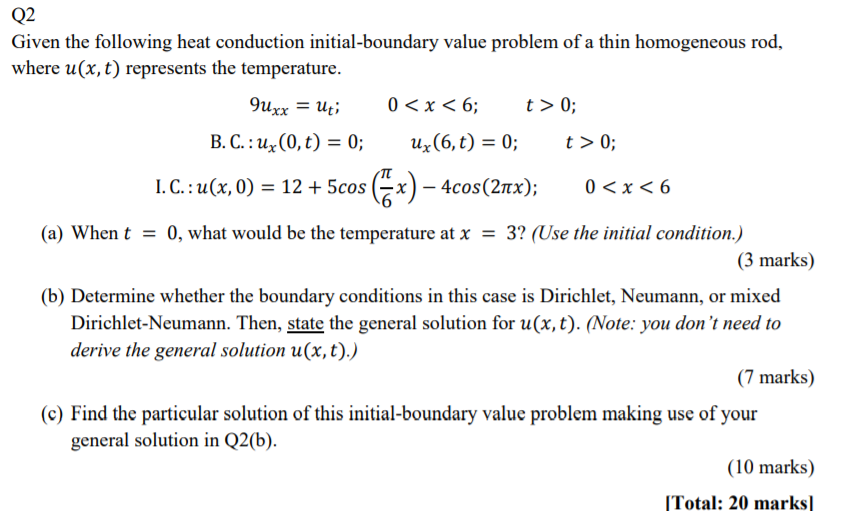Q2 Given the following heat conduction initial-boundary value problem of a thin homogeneous rod, where ?(?,?) represents the temperature. 9??? = ?? ; 0 < ? < 6; ? > 0; B. C. : ?? (0,?) = 0; ?? (6,?) = 0; ? > 0; I. C. : ?(?, 0) = 12 + 5??? ( ? 6 ?) − 4???(2??); 0 < ? < 6 (a) When ? = 0, what would be the temperature at ? = 3? (Use...

• ### Q2 Given the following heat conduction initial-boundary value problem of a thin homogeneous rod, where u(x,...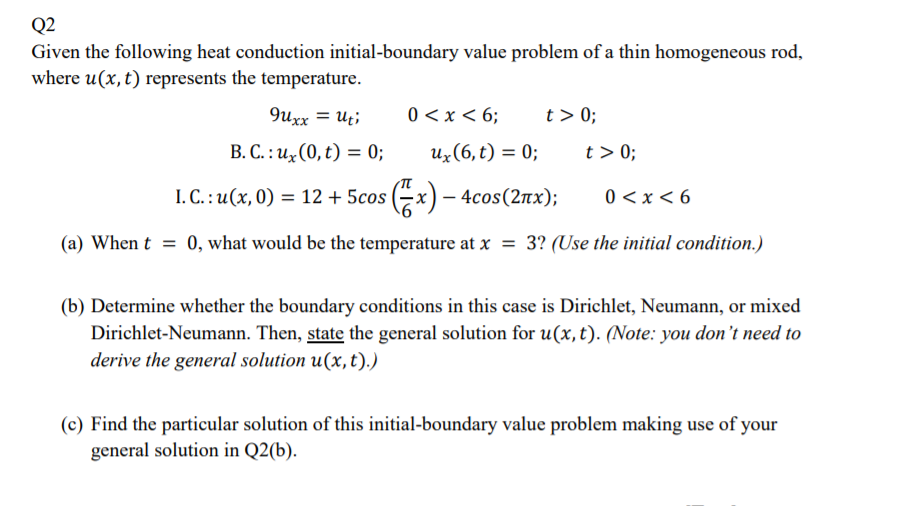Q2 Given the following heat conduction initial-boundary value problem of a thin homogeneous rod, where u(x, t) represents the temperature. 9uxx = ut; 0 < x < 6; t> 0; B.C.: ux(0,t) = 0; uz(6,t) = 0; t>0; I. C.: u(x,0) = 12 + 5cos (6x) – 4cos(21x); 0<x< 6 (a) When t = 0, what would be the temperature at x = 3? (Use the initial condition.) (b) Determine whether the boundary conditions in this case is Dirichlet, Neumann,...

• ### Q2 Given the following heat conduction initial-boundary value problem of a thin homogeneous rod, where u(x,...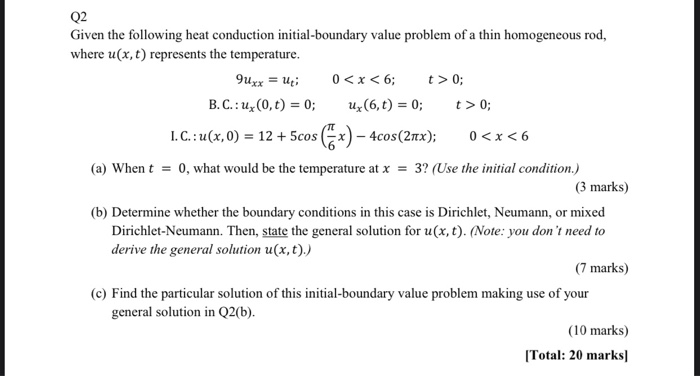Q2 Given the following heat conduction initial-boundary value problem of a thin homogeneous rod, where u(x, t) represents the temperature. 9uxx = uti 0 < x < 6; t>0; B.C.: 4x(0,t) = 0; uz (6,t) = 0; t> 0; 1. C.: u(x,0) = 12 + 5cos (6x) – 4cos(27x); 0<x<6 (a) When t = 0, what would be the temperature at x = 3? (Use the initial condition.) (3 marks) (b) Determine whether the boundary conditions in this case is...